### Home > CALC > Chapter 7 > Lesson 7.2.4 > Problem7-97

7-97.
1. Find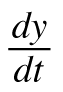for each of the following functions. Homework Help ✎

1.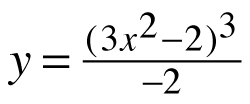2. y = 6(3x2 − 2)3

3. (2y)2 − cos(x5) = 9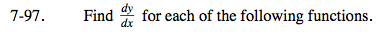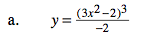This is NOT a Quotient Rule problem!

$\text{Before differentiating, rewrite the equation as }y=-\frac{1}{2}(3x^{2}-2)^{3}.$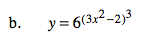= 18ln(6)x(3x2 − 2)2 6(3x2 − 2)3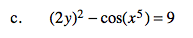Use implicit differentiation.